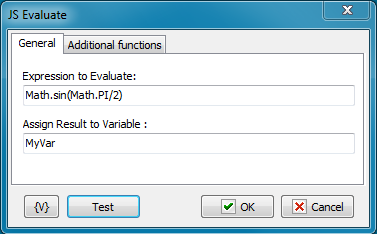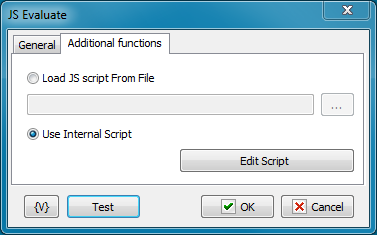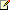﻿ JS Evaluate - RoboTask User's Guide
 This action allows to calculate an expression value and then save it into a variable. The expression has to be written according to the syntax of JS language. The documentation for the language can be read on the Microsoft site: http://msdn.microsoft.com/en-us/library/hbxc2t98(v=vs.84).aspx The action also allows to connect the function library from an external file or directly in an additional script. For example, try to calculate the expression sum(5,6) + multi(3,4) And we get the error. The JavaScript language doesn't contain functions sum and multi. To solve this, it is possible to specify a little library of user functions: function sum(a, b) {  return a+b; }   function multi(a, b) {  return a*b; } In this case the action calculates the result without any troubles. The given example is too simple and is cited for the demonstration solely.   Another example. Calculate the factorial of a number. Such function is absent in VB Script. But it is easy to evade. It takes only to create the simply function: function factorial(a)  {  if (a>30)    { //this is synthetic limitation      throw new Error(1, "Parameter more than 30");    }  res = 1;  for (i=1; i<=a; i++)    {      res *= i;    }  return res;  }   Now we easy can calculate the expression: factorial(15) Given possibility allows to do some specific calculations, that are difficult to be written in one line.   General TabExpression To Evaluate Write down the expression whose value needs to be calculated. The expression has to be made according to the syntax of JavaScript.   Assign Result to Variable Enter the name of the variable into which it is necessary to save the result.   Additional FunctionsLoad JS Script from File Load the library of additional functions from an external file. Enter a file name or choose it interactively. The file name can be empty. In this case the action works only with standard functions  and objects of JS.   Use Internal Script If you want to specify functions directly in a task, choose this option. To edit a script, click the button Edit Script. You can see the editor like in the action JS Script. The text of a script can be empty. In this case the action works only with standard functions  and objects of JS.Related Topics Script Extensions JS script VB Script VB Evaluate RoboTaskApp object